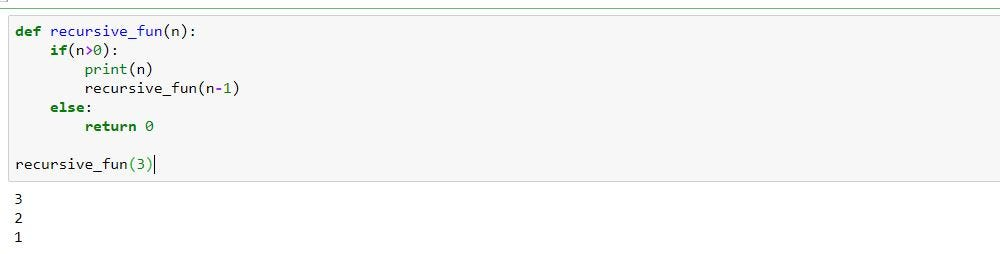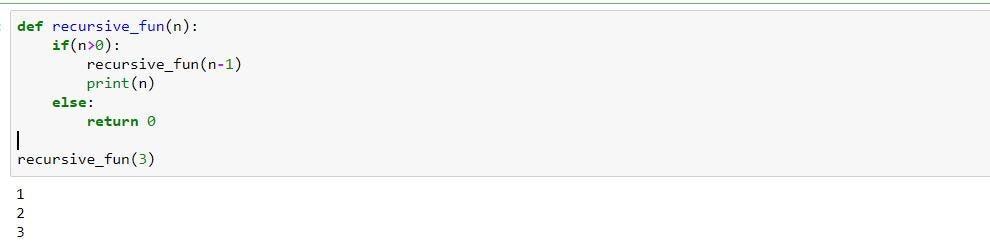1603760400

# Introduction to Recursive CTE

This article will introduce the concept of SQL recursive. Recursive CTE is a really cool. We will see that it can often simplify our code, and avoid a cascade of SQL queries!

### Why use a recursive CTE ?

The recursive queries are used to query hierarchical data. It avoids a cascade of SQL queries, you can only do one query to retrieve the hierarchical data.

### What is recursive CTE ?

First, what is a CTE? A CTE (Common Table Expression) is a temporary named result set that you can reference within a SELECT, INSERT, UPDATE, or DELETE statement. For example, you can use CTE when, in a query, you will use the same subquery more than once.

A recursive CTE is one having a subquery that refers to its own name!

Recursive CTE is defined in the SQL standard.

### How to make a recursive CTE?

A recursive CTE has this structure:

• The WITH clause must begin with “WITH RECURSIVE”
• The recursive CTE subquery has two parts, separated by “UNION [ALL]” or “UNION DISTINCT”:
• The first part produces the initial row(s) for the CTE. This SELECT does not refer to the CTE name.
• The second part recurses by referring to the CTE name in its FROM clause.

### Practice / Example

In this example, we use hierarchical data. Each row can have zero or one parent. And it parent can also have a parent etc.

``````Create table test (id integer, parent_id integer);

insert into test (id, parent_id) values (1, null);

insert into test (id, parent_id) values (11, 1);
insert into test (id, parent_id) values (111, 11);

insert into test (id, parent_id) values (112, 11);

insert into test (id, parent_id) values (12, 1);

insert into test (id, parent_id) values (121, 12);
``````

For example, the row with id 111 has as ancestors: 11 and 1.

Before knowing the recursive CTE, I was doing several queries to get all the ancestors of a row.

For example, to retrieve all the ancestors of the row with id 111.

``````While (has parent)

Select id, parent_id from test where id = X
``````

With recursive CTE, we can retrieve all ancestors of a row with only one SQL query :)

``````WITH RECURSIVE cte_test AS (
SELECT id, parent_id FROM test WHERE id = 111
UNION
SELECT test.id, test.parent_id FROM test JOIN cte_test ON cte_test.id = test.parent_id
``````

`) SELECT * FROM cte_test`

Explanations:

• “WITH RECURSIVE”:

It indicates we will make recursive

• “SELECT id, parent_id FROM test WHERE id = 111”:

It is the initial query.

• “UNION … JOIN cte_test” :

It is the recursive expression! We make a jointure with the current CTE!

Replay this example here

#sql #database #sql-server #sql-injection #writing-sql-queries #sql-beginner-tips #better-sql-querying-tips #sql-top-story

## Buddha Community1603537200

## While You Don't Understand Recursion, Read Recursion: by Randy Taylor

Recursion is the one idea I constantly use while I solve coding problems. Most of the time I don’t start by thinking “RECURSION WILL SOLVE THIS!”. However recursion just ends up being the logical way to reach an answer. In my professional opinion recursion is the purest form of coding; write a function that will call itself until you get what you want! To implement recursion we will create a helper algorithm. 1) Identify what the smallest input is. 2) Continually break down a larger input into smaller inputs and pass those smaller inputs back into itself until you get the desired answer. 3) Define a “base case” that will stop the Recursion should the answer not be found.

Let’s look at the idea of Recursion first. We are writing code that will execute itself until we get a desired answer or reach a base case. Essentially we are creating a loop. I will illustrate this with pseudo code:

``````for (let recursion =()=>{ …answer? answer = true : false} ; answer === false; recursion())
``````

Much like a traditional for loop the above pseudo code will continue while the second condition is true; the recursion will continue until answer === true. At this point the second statement of the for loop is false terminating the loop. Above if answer === false recursion will call itself again. This is the general idea of recursion. This is why creating a base case is essential to prevent an infinite loop. The “answer” we are looking for might not be present causing recursion to run until the sun burns out.

#algorithms #javascript #recursion #tutorial-for-beginners #iteration #recursion-explained #what-is-recursion #programming1621308160

## Using the PostgreSQL Recursive CTE – Part Two

This is the second of a two-part blog series. Because a hierarchy is the most restrictive kind of graph, [Part One]  used a simple representation and a simple traversal method. The Bacon Numbers problem is based on the most general kind of graph—an undirected cyclic graph . Part Two therefore uses the general graph representation and the general traversal method.

[Using a recursive CTE to compute Bacon Numbers for actors listed in the IMDb]
All the code snippets and pictures in this post are copied from the YSQL documentation. The main page of the overall [WITH] [** clause and common table expressions**] section explains that all the code is available in a downloadable code zip with a few master scripts to run all of the code mechanically.

#postgresql #recursive #cte #imdb #bacon numbers1621307513

## Using the PostgreSQL Recursive CTE – Part One

This is the first post of a two-part blog series. Each of these case studies, the employee hierarchy and the [Bacon Numbers problem] , is a famous example of the use of the PostgreSQL recursive common table expression (CTE). Because [YugabyteDB re-uses the PostgreSQL SQL processing code]  “as is” , everything that I say in the present two-part blog post series applies in the same way to PostgreSQL as it does to YugabyteDB.

[ ﻿Using a recursive CTE to compute Bacon Numbers for actors listed in the IMDb]
All the code snippets and pictures in this post are copied from the YSQL documentation. The main page of the overall  [WITH] [** clause and common table expressions**] section explains that all the code is available in a downloadable code zip with a few master scripts to run all of the code mechanically.

#postgresql #recursive #cte1599158640

Anybody reading data structures and algorithm would have come across a topic called “Recursion”. Now here is the best way to understand recursion. Read this ’n’ times until your understanding becomes** “True”**. Haha!! now that’s how simple the recursion is.

Well recursion can be implemented in any language and it has the same outcome. Here i’ll be demonstrating few topics and key concepts on recursion in python🐍. Yes, you read it right one love for python❤.

## Introduction:

A function calling itself until a condition is set true or false is called recursive function. The below image shows to a simple function called “recursive_fun” calling itself ’n’ times.a simple example of recursion

The output of a recursion can be understood by a concept called as tracing which is done to trace the output by a recursive function.

a tracing tree of recursive_fun(forward phase)

the above tracing tree explains a lot about how the repetitive function call takes place to obtain the desired output. Here the recursive_fun is called 3+1 times where for all the 3 times it prints ’n’ but for the last call it fails to satisfy the condition and returns 0. Hence, in any recursion the function call is made n+1 times. Also, on carefully observing the tracing tree we see that the recursive_fun executes print statement before calling itself, this is known as forward phase. A backward phase of recursion is when a function calls itself before executing any statements, i.e all the other statements are executed until there is no further function calls.#recursive #algorithms #python #recursion #data-structures1594369800

## Introduction to Structured Query Language SQL pdf

SQL stands for Structured Query Language. SQL is a scripting language expected to store, control, and inquiry information put away in social databases. The main manifestation of SQL showed up in 1974, when a gathering in IBM built up the principal model of a social database. The primary business social database was discharged by Relational Software later turning out to be Oracle.

Models for SQL exist. In any case, the SQL that can be utilized on every last one of the major RDBMS today is in various flavors. This is because of two reasons:

1. The SQL order standard is genuinely intricate, and it isn’t handy to actualize the whole standard.

2. Every database seller needs an approach to separate its item from others.

Right now, contrasts are noted where fitting.#programming books #beginning sql pdf #commands sql #download free sql full book pdf #introduction to sql pdf #introduction to sql ppt #introduction to sql #practical sql pdf #sql commands pdf with examples free download #sql commands #sql free bool download #sql guide #sql language #sql pdf #sql ppt #sql programming language #sql tutorial for beginners #sql tutorial pdf #sql #structured query language pdf #structured query language ppt #structured query language